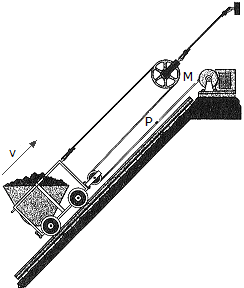# Engineering Mechanics - Kinematics of Particle (KOP) - Discussion

### Discussion :: Kinematics of Particle (KOP) - General Questions (Q.No.22)

22.The mine car is being pulled up to the inclined plane using the motor M and the rope-and-pulley arragement shown. Determine the speed vp at which a point P on the cable must be traveling toward the motor to move the the car up the plane with a constant speed of v = 5 m/s.

 [A]. VP = 1.667 m/s [B]. VP = 2.50 m/s [C]. VP = 15.00 m/s [D]. VP = 10.00 m/s

Explanation:

No answer description available for this question.

 Jorge Oberdan said: (Aug 22, 2012) 2Sc +(Sc - Sp) = l 3Sc - vp = 0; vp = 3*(-5) = -15m/s = 15m/s